Test: Phase Controlled Rectifiers- 2

# Test: Phase Controlled Rectifiers- 2

Test Description

## 15 Questions MCQ Test GATE Electrical Engineering (EE) 2023 Mock Test Series | Test: Phase Controlled Rectifiers- 2

Test: Phase Controlled Rectifiers- 2 for Electrical Engineering (EE) 2023 is part of GATE Electrical Engineering (EE) 2023 Mock Test Series preparation. The Test: Phase Controlled Rectifiers- 2 questions and answers have been prepared according to the Electrical Engineering (EE) exam syllabus.The Test: Phase Controlled Rectifiers- 2 MCQs are made for Electrical Engineering (EE) 2023 Exam. Find important definitions, questions, notes, meanings, examples, exercises, MCQs and online tests for Test: Phase Controlled Rectifiers- 2 below.
Solutions of Test: Phase Controlled Rectifiers- 2 questions in English are available as part of our GATE Electrical Engineering (EE) 2023 Mock Test Series for Electrical Engineering (EE) & Test: Phase Controlled Rectifiers- 2 solutions in Hindi for GATE Electrical Engineering (EE) 2023 Mock Test Series course. Download more important topics, notes, lectures and mock test series for Electrical Engineering (EE) Exam by signing up for free. Attempt Test: Phase Controlled Rectifiers- 2 | 15 questions in 45 minutes | Mock test for Electrical Engineering (EE) preparation | Free important questions MCQ to study GATE Electrical Engineering (EE) 2023 Mock Test Series for Electrical Engineering (EE) Exam | Download free PDF with solutions
 1 Crore+ students have signed up on EduRev. Have you?
Test: Phase Controlled Rectifiers- 2 - Question 1

### Consider the following statements associated with single phase full converters: 1. Mid-point converter configuration is used in case the terminals on dc side have to be grounded. 2. The transformer rating in mid-point converter is double the load rating. 3. SCRs are subjected to a peak inverse voltage of 2 Vm in single phase mid-point converter. 4. Bridge converter is preferred over mid-point converter. Which of these statements are correct?

Detailed Solution for Test: Phase Controlled Rectifiers- 2 - Question 1

In mid-point converter, each secondary should be able to supply the load power. Due to this reason, the transformer rating in mid-point converter is double the load rating. Thus, statement-2 is not correct.

Test: Phase Controlled Rectifiers- 2 - Question 2

### Assertion (A): The commutation overlap is more predominant is semiconverters than in full converters. Reason (R): The commutation period in seconds, when outgoing and incoming SCRs are conducting, is known as the overlap period.

Detailed Solution for Test: Phase Controlled Rectifiers- 2 - Question 2

The commutation overlap is more predominant in full converter than in semi converter. Hence, assertion is a false statement.

Test: Phase Controlled Rectifiers- 2 - Question 3

### For a single-phase full converter having a full load current of I0 match List - I (Overlap angle) with List-ll (Output voltage) and select the correct answer using the codes given below the lists (Ls = source inductance):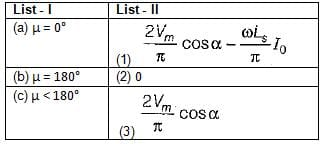Codes: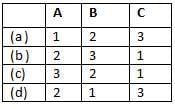Detailed Solution for Test: Phase Controlled Rectifiers- 2 - Question 3

• In a single-phase full converter, as long as μ < 180°, the output voltage will be given by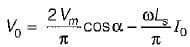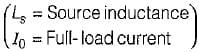• When μ = 180°, the load will be permanently short circuited by SCRs and the output voltage will be zero because during the overlap angle, all SCRs will be conducting.
•  Since,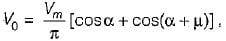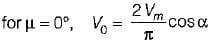Test: Phase Controlled Rectifiers- 2 - Question 4

Which of the following converters can feed power in any of the four quadrants?

Detailed Solution for Test: Phase Controlled Rectifiers- 2 - Question 4

A dual converter using two full converters can give four quadrant operation as shown below.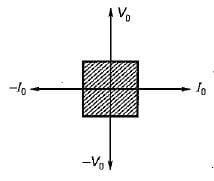Test: Phase Controlled Rectifiers- 2 - Question 5

Consider the following statements:
1. In a full converter, direction of current cannot reverse.
2. Semi-converters are single quadrant converters.
3. A full converter can operate as a two-quadrant converter.
4. A full converter operates as a rectifier in first quadrant and as an inverter in the second quadrant.
5. In a full converter, direction of current cannot reverse but polarity of output voltage can be reversed.

Q. Which of the statements given above are correct?

Detailed Solution for Test: Phase Controlled Rectifiers- 2 - Question 5

A full converter operates as a rectifier in first quadrant (both V0, I0 positive) from α = 0° to 90° and as an inverter (V0 positive but I0 negative) from α = 90° to 180° in the fourth quadrant as shown below.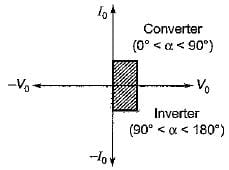Thus, statement-4 is not correct. Statements 1, 2,3 and 5 are correct.

Test: Phase Controlled Rectifiers- 2 - Question 6

AC-to-DC circulating current dual converters are operated with which of the following relationship between their triggering angles (α1 and α2)?

Detailed Solution for Test: Phase Controlled Rectifiers- 2 - Question 6

In AC-to-DC circulating current dual converters, the firing pulses of the two converters are so adjusted that α1 + α2 = 180°.

Test: Phase Controlled Rectifiers- 2 - Question 7

A single-phase half-wave controlled converter with resistive load is operated from a 120 V, 50 Hz supply. If the average output voltage is 25% of the maximum possible output voltage, the firing angle of the thyristor would be equal to

Detailed Solution for Test: Phase Controlled Rectifiers- 2 - Question 7

The average output voltage is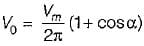The maximum output voltage is obtained when α = 0°
∴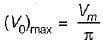According to question,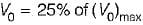or,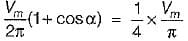or,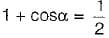or,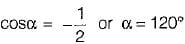Test: Phase Controlled Rectifiers- 2 - Question 8

A single phase fully controlled thyristor bridge converter supplies a load consisting of R, L and E has shown in figure below. The inductance L in the circuit is so large that the output current may be considered to be virtually constant. The firing angle α is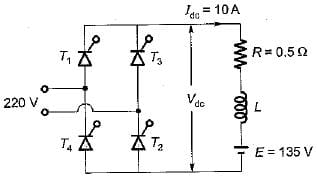Detailed Solution for Test: Phase Controlled Rectifiers- 2 - Question 8

For a single-phase fully controlled thyristor bridge converter with R, L, E load, the average output voltage is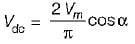or,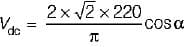= 198 cos α ....(i)
Now, Vdc - Idc R+ E- (10 x 0.5) + 135
or, Vdc = 140V    ...(ii)
From equations (i) and (ii),we have:
198 cos α = 140
or,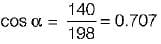or, Firing angle α = 45°

Test: Phase Controlled Rectifiers- 2 - Question 9

Consider the following statements:
1. Fully-controlled and half controlled circuits both are capable of regeneration.
2. The higher the pulse number, the lower is the magnitude of ripple voltage and current in the load-circuit and the a.c. supply.
3. Bridge circuits and half-wave circuits both require same number of thyristors to carry the same current.
4. Half-wave circuits tend to be used for low-voltage, high-current use.Which of the statements given above is/are not correct?

Detailed Solution for Test: Phase Controlled Rectifiers- 2 - Question 9

• Only fully controlled circuits are capable of inversion and therefore regeneration, while half-controlled circuits are not. Thus, statement-1 is not correct.
• Statement-2 is correct.
• Bridge circuits require twice as many thyristors than half-wave circuits to carry the same current. Thus, statement-3 is not correct.
• Statement-4 is correct.

Test: Phase Controlled Rectifiers- 2 - Question 10

In a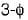bridge converter the dc load current is 90 A, the commutating inductance per phase is 50 and thepower supply is 2.3 kV, 50 Hz. The reduction in output voltage due to overlap is around

Detailed Solution for Test: Phase Controlled Rectifiers- 2 - Question 10

The reduction in output voltage due to overlap is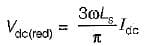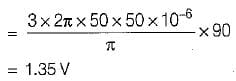Test: Phase Controlled Rectifiers- 2 - Question 11

The holding current of SCRs in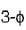full-converter is 200 mA and the delay time is 2.5 p.sec. Converter is supplied from a star-connected 208 V, 50 Hz supply, has a load of R = 2 Ω L = 8 mH and the firing angle is 60°. The minimum width of gate pulse tp for L = 0 H is

Detailed Solution for Test: Phase Controlled Rectifiers- 2 - Question 11

Given,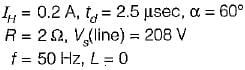∴ Phase voltage,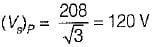and peak voltage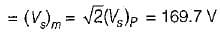Now,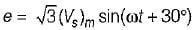At ωt = 60°, e = √3x169.7sin90° ≈ 293.93V
Rate of rise of anode current, di/dt at the instant of triggering is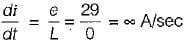∴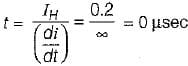∴ Minimum width of gate-pulse is
tp = t + td = 0 + 2.5 = 2.5μs

Test: Phase Controlled Rectifiers- 2 - Question 12

The nature of load current, l.e., whether load is continuous or discontinuous in controlled rectifiers

Detailed Solution for Test: Phase Controlled Rectifiers- 2 - Question 12

The term continuous means that load current never ceases but continues to flow through SCR or their combination. The term discontinuous is applied to the condition when load current reaches zero during each half cycle before the next SCR in sequence is fired. In practice, the output current may become discontinuous at high values of firing angle or at low values of load current.

Test: Phase Controlled Rectifiers- 2 - Question 13

In a 3-phase full converter, the output voltage during overlap is equal to

Detailed Solution for Test: Phase Controlled Rectifiers- 2 - Question 13

Output voltage with no overlap = internal voltage of the 3-phase full converter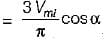Output voltage with overlap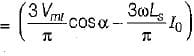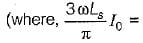Average value of fall in output voltage due to overlap)

Test: Phase Controlled Rectifiers- 2 - Question 14

The peak inverse voltage in ac to dc converter system is highest in

Detailed Solution for Test: Phase Controlled Rectifiers- 2 - Question 14

PIV of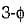bridge converter =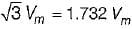PIV ofhalf wave converter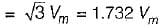PIV of single-phase full-wave mid-point converter
= - 2 Vm (Highest)
PIV of single-phase full-converter = - Vm

Test: Phase Controlled Rectifiers- 2 - Question 15

A transformer is having source voltage of 400 volt and turns ratio of 2:1. The transformer is centre-tapped. If the secondary is connected to single phase full-wave converter, PIV per SCR will be

Detailed Solution for Test: Phase Controlled Rectifiers- 2 - Question 15

Secondary voltage =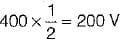∴ PIV = 200√2 V

## GATE Electrical Engineering (EE) 2023 Mock Test Series

21 docs|273 tests
 Use Code STAYHOME200 and get INR 200 additional OFF Use Coupon Code
Information about Test: Phase Controlled Rectifiers- 2 Page
In this test you can find the Exam questions for Test: Phase Controlled Rectifiers- 2 solved & explained in the simplest way possible. Besides giving Questions and answers for Test: Phase Controlled Rectifiers- 2, EduRev gives you an ample number of Online tests for practice

## GATE Electrical Engineering (EE) 2023 Mock Test Series

21 docs|273 tests